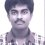# Help: Functional Equation

If $f(x)$ is a polynomial in $x$ satisfying $f(x) f(y) + 2 = f(x) + f(y) + f(xy)$ and $f(0) = 0, f'(1) = 2$, find $f(x)$.4 years, 7 months ago

This discussion board is a place to discuss our Daily Challenges and the math and science related to those challenges. Explanations are more than just a solution — they should explain the steps and thinking strategies that you used to obtain the solution. Comments should further the discussion of math and science.

When posting on Brilliant:

• Use the emojis to react to an explanation, whether you're congratulating a job well done , or just really confused .
• Ask specific questions about the challenge or the steps in somebody's explanation. Well-posed questions can add a lot to the discussion, but posting "I don't understand!" doesn't help anyone.
• Try to contribute something new to the discussion, whether it is an extension, generalization or other idea related to the challenge.

MarkdownAppears as
*italics* or _italics_ italics
**bold** or __bold__ bold
- bulleted- list
• bulleted
• list
1. numbered2. list
1. numbered
2. list
Note: you must add a full line of space before and after lists for them to show up correctly
paragraph 1paragraph 2

paragraph 1

paragraph 2

[example link](https://brilliant.org)example link
> This is a quote
This is a quote
    # I indented these lines
# 4 spaces, and now they show
# up as a code block.

print "hello world"
# I indented these lines
# 4 spaces, and now they show
# up as a code block.

print "hello world"
MathAppears as
Remember to wrap math in $$ ... $$ or $ ... $ to ensure proper formatting.
2 \times 3 $2 \times 3$
2^{34} $2^{34}$
a_{i-1} $a_{i-1}$
\frac{2}{3} $\frac{2}{3}$
\sqrt{2} $\sqrt{2}$
\sum_{i=1}^3 $\sum_{i=1}^3$
\sin \theta $\sin \theta$
\boxed{123} $\boxed{123}$

Sort by:

Is the question all right?? Put y=0 in the given functional equation to get: $0+2=f(x)+0+0~~~~\small{\text{(since f(0)=0)}}$ $\Large f(x)=2$ which does not satisfy the condition f'(1)=2 !!

(It might work if indeed it was given f(1)=2 and f'(0)=0)

- 4 years, 7 months ago

I also came up with the same conclusion as you, i.e. $f(x)=2$, so i thought of using the $f'(1)=2$. So as we know from the definition of derivatives

$f'(1)=\frac { f(1+h)-f(1) }{ h }\\$

$\Longrightarrow f'(1)=\frac { f(1(1+h))-f(1) }{ h }$

From the functional equation we have,$\\$

$f(1)f(1+h))+2=f(1)+f(1+h)+f(1(1+h))$

$\Longrightarrow f(1+h)=f(1)f(1+h)-f(1)-f(1+h)+2$

$\therefore \quad f^{ 1 }\left( x \right) =\frac { f(1)f(1+h)-f(1)-f(1+h)+2-f(1) }{ h } =\frac { f(1)(f(1+h)-2)-(f(1+h)-2) }{ h }$

$\Longrightarrow f^{ 1 }\left( x \right) =\frac { (f(1)-1)(f(1+h)-2) }{ h }$

$\Longrightarrow 2h=(f(1)-1)(f(1+h)-2)$

From here on I am not able to conclude anything useful, so if you would help me lead in the right direction or tell me something that i am doing wrong.

- 4 years, 7 months ago

You have to take the limit for the derivative, as h goes to 0.

- 4 years, 7 months ago

Please if you can post a solution@parv mor

- 4 years, 7 months ago

f(1)=2 and f'(0)=0 should be there for the question to be correct

- 4 years, 7 months ago

But your suggestion makes the functional equation pretty much useless, as for $x=1$, and $y=c$, $c\in dom(f)$ we do not get any relation to solve for $f(x)$

- 4 years, 7 months ago

Ya.. I also reached the same conclusion...

- 4 years, 7 months ago

Hey, if you see the other post, which is the same question, here,
you'll see we get,
$f(x) - 1 = x^t$
For no value of t, will both the given conditions be satisfied- there is no such function, as a result. Try it out! I think you should check your question again.

- 4 years, 7 months ago

The question is wrong . I will prove it . Put $x,y=0$ $f(0)^{2}-3f(0)+2=0$ This implies $f(0)=1,2$ now put $x,y=1$ this gives $f(1)=1,2$ Differentiating the function partially with respect to $x$ we get $f'(x)f(y)=f'(x)+yf'(xy).......(1)$ Now put $x,y=1$ in $(1)$ We get $f(1)=2$ Now put $x,y=0$ in $(1)$ We get$f(0)=1..or..f'(0)=0$ Now put $x=1$ in (1) we get $\frac{2f(y)-2}{y}=f'(y)...(2)$ Now let $f(0)=1$ , we have $f(1)=2$then we observe that $f(x)=x+1$ also in satisfies main functional equation and (2),(1) but $f'(x)=1$ hence question is wrong . If only main functional equation is given without any condition then $f(x)=x+1$.

- 4 years, 7 months ago